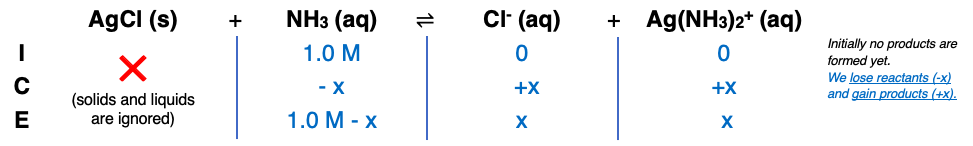# Problem: Kf for the complex ion Ag(NH3)2+ is 1.7 X 107. Ksp for AgCl is 1.6 X 10 -10. Calculate the molar solubility of AgCl in 1.0 M NH3.

###### FREE Expert Solution

Step 1:Step 2:

AgCl (s)  Ag+ (aq) + Cl- (aq)

Ag+ (aq) + 2 NH3+ (aq)  [Ag(NH3)2+] (aq)

AgCl (s) + NH3 (aq)  Cl- (aq) + Ag(NH3)2+ (aq)

96% (243 ratings)###### Problem Details

Kf for the complex ion Ag(NH3)2+ is 1.7 X 107. Ksp for AgCl is 1.6 X 10 -10. Calculate the molar solubility of AgCl in 1.0 M NH3.

Frequently Asked Questions

What scientific concept do you need to know in order to solve this problem?

Our tutors have indicated that to solve this problem you will need to apply the Formation Constant concept. If you need more Formation Constant practice, you can also practice Formation Constant practice problems.

What professor is this problem relevant for?

Based on our data, we think this problem is relevant for Professor Carlon's class at UCB.

What textbook is this problem found in?

Our data indicates that this problem or a close variation was asked in Chemistry: An Atoms First Approach - Zumdahl Atoms 1st 2nd Edition. You can also practice Chemistry: An Atoms First Approach - Zumdahl Atoms 1st 2nd Edition practice problems.